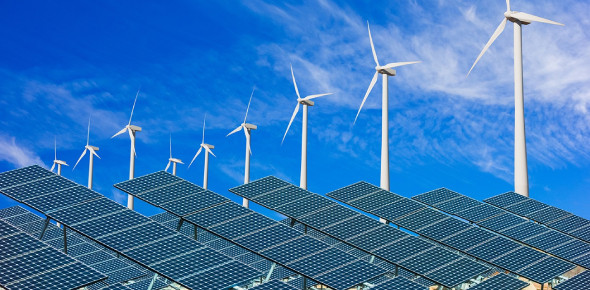# Energy Resources Quiz! MCQ Trivia Facts!

30 Questions | Attempts: 2504
ShareSettingsEnergy resources quiz MCQ trivia facts! Energy is the ability to do work, and there are many ways that we get energy. Energy resources are basically mattered, that is a source of energy. These sources can either be renewable or non-renewable. The different sources of energy can either be renewable, fossil or nuclear. This quick quiz is designed to help you review how much you know about them. Do give it a shot!

• 1.
The burning of fossil fuels produces carbon dioxide that increases global warming.
• A.

True

• B.

False

• 2.
Solar energy and wind energy are examples of inexhaustible energy resources.
• A.

True

• B.

False

• 3.
Nuclear energy is fossil fuel.
• A.

True

• B.

False

• 4.
Coal, natural gas, and petroleum are fossil fuels.
• A.

True

• B.

False

• 5.
Geothermal energy comes from underground pockets of hot water and steam.
• A.

True

• B.

False

• 6.
Energy resources that are nonrenewable cannot be used up.
• A.

True

• B.

False

• 7.
Energy conservation is important in helping us reduce the rate at which we use ______________ energy resources. (renewable or nonrenewable)
• 8.
Which energy source makes gasoline, fuel, oil, diesel, and kerosene?
• A.

Coal

• B.

Nuclear

• C.

Natural Gas

• D.

Petroleum

• 9.
Which energy source uses the kinetic energy from falling water?
• A.

Geothermal

• B.

Hydroelectric

• C.

Biomass

• D.

Solar

• 10.
Which energy source is wood considered?
• A.

Coal

• B.

Biomass

• C.

Geothermal

• D.

Petroleum

• 11.
Which energy source is fossil fuel which produces the least amount of pollution?
• A.

Coal

• B.

Nuclear

• C.

Natural Gas

• D.

Petroleum

• 12.
Which energy source uses uranium?
• A.

Coal

• B.

Nuclear

• C.

Natural Gas

• D.

Petroleum

• 13.
Which energy source is blackish rock formed millions of year ago?
• A.

Coal

• B.

Nuclear

• C.

Natural Gas

• D.

Petroleum

• 14.
Which energy source uses turbines to transform kinetic energy into electrical energy?
• A.

Geothermal

• B.

Wind

• C.

Biomass

• D.

Solar

• 15.
Which energy source is thermal energy inside Earth?
• A.

Geothermal

• B.

Wind

• C.

Biomass

• D.

Solar

• 16.
Which energy source is radiant energy from the sun?
• A.

Geothermal

• B.

Hydroelectric

• C.

Biomass

• D.

Solar

• 17.
Choose the energy resources that are nonrenewable.
• A.

Natural Gas

• B.

Geothermal

• C.

Nuclear

• D.

Wind

• E.

Solar

• F.

Coal

• G.

Petroleum

• H.

Hydroelectric

• I.

Biomass

• 18.
Choose the energy resources that are renewable.
• A.

Natural Gas

• B.

Geothermal

• C.

Nuclear

• D.

Wind

• E.

Solar

• F.

Coal

• G.

Petroleum

• H.

Hydroelectric

• I.

Biomass

• 19.
Almost all the energy in foods and fuels can be traced to energy from inside Earth.
• A.

True

• B.

False

• 20.
A geothermal power plant transforms the kinetic energy in moving water into electrical energy.
• A.

True

• B.

False

• 21.
Fossil fuels are nonrenewable energy resources.
• A.

True

• B.

False

• 22.
An inexhaustible energy resource is an energy source that cannot be used up.
• A.

True

• B.

False

• 23.
Conserving energy reduces the rate at which energy resources are used.
• A.

True

• B.

False

• 24.
A disadvantage of using nuclear energy is air pollution.
• A.

True

• B.

False

• 25.
A renewable energy resource is an energy source that is replaced as fast, or faster than it is used.
• A.

True

• B.

False

## Related TopicsBack to top
×

Wait!
Here's an interesting quiz for you.